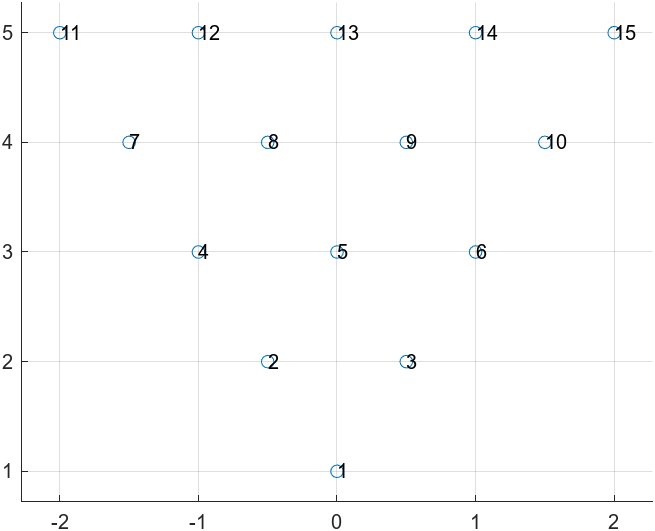# Problem 56200. Triangle Coordinates

Given a natural number n, return two-element vectors, x and y, containing the coordinates of a triangular arrangement of points, where:
• there are n rows of points
• the kth row contains k points
• all points in the kth row have y = k
• the x coordinates in each row are equally spaced a distance of 1 and centered at 0
The points should be given in order, row 1 to row n, with the x coordinate in each row given in ascending numerical order.
For example:x = [0 -0.5 0.5 -1 0 1 -1.5 -0.5 0.5 1.5 -2 -1 0 1 2]
y = [1 2 2 3 3 3 4 4 4 4 5 5 5 5 5]

### Solution Stats

73.64% Correct | 26.36% Incorrect
Last Solution submitted on Dec 07, 2023

### Community Treasure Hunt

Find the treasures in MATLAB Central and discover how the community can help you!

Start Hunting!